# Fraction calculator

The calculator performs basic and advanced operations with fractions, expressions with fractions combined with integers, decimals, and mixed numbers. It also shows detailed step-by-step information about the fraction calculation procedure. Solve problems with two, three, or more fractions and numbers in one expression.

## Result:

### (3/4) : (5/7) = 21/20 = 1 1/20 = 1.05

Spelled result in words is twenty-one twentieths (or one and one twentieth).

### How do you solve fractions step by step?

1. Divide: 3/4 : 5/7 = 3/4 · 7/5 = 3 · 7/4 · 5 = 21/20
Dividing two fractions is the same as multiplying the first fraction by the reciprocal value of the second fraction. The first sub-step is to find the reciprocal (reverse the numerator and denominator, reciprocal of 5/7 is 7/5) of the second fraction. Next, multiply the two numerators. Then, multiply the two denominators. In the next intermediate step, the fraction result cannot be further simplified by canceling.
In words - three quarters divided by five sevenths = twenty-one twentieths.

#### Rules for expressions with fractions:

Fractions - use the slash “/” between the numerator and denominator, i.e., for five-hundredths, enter 5/100. If you are using mixed numbers, be sure to leave a single space between the whole and fraction part.
The slash separates the numerator (number above a fraction line) and denominator (number below).

Mixed numerals (mixed fractions or mixed numbers) write as non-zero integer separated by one space and fraction i.e., 1 2/3 (having the same sign). An example of a negative mixed fraction: -5 1/2.
Because slash is both signs for fraction line and division, we recommended use colon (:) as the operator of division fractions i.e., 1/2 : 3.

Decimals (decimal numbers) enter with a decimal point . and they are automatically converted to fractions - i.e. 1.45.

The colon : and slash / is the symbol of division. Can be used to divide mixed numbers 1 2/3 : 4 3/8 or can be used for write complex fractions i.e. 1/2 : 1/3.
An asterisk * or × is the symbol for multiplication.
Plus + is addition, minus sign - is subtraction and ()[] is mathematical parentheses.
The exponentiation/power symbol is ^ - for example: (7/8-4/5)^2 = (7/8-4/5)2

#### Examples:

adding fractions: 2/4 + 3/4
subtracting fractions: 2/3 - 1/2
multiplying fractions: 7/8 * 3/9
dividing Fractions: 1/2 : 3/4
exponentiation of fraction: 3/5^3
fractional exponents: 16 ^ 1/2
adding fractions and mixed numbers: 8/5 + 6 2/7
dividing integer and fraction: 5 ÷ 1/2
complex fractions: 5/8 : 2 2/3
decimal to fraction: 0.625
Fraction to Decimal: 1/4
Fraction to Percent: 1/8 %
comparing fractions: 1/4 2/3
multiplying a fraction by a whole number: 6 * 3/4
square root of a fraction: sqrt(1/16)
reducing or simplifying the fraction (simplification) - dividing the numerator and denominator of a fraction by the same non-zero number - equivalent fraction: 4/22
expression with brackets: 1/3 * (1/2 - 3 3/8)
compound fraction: 3/4 of 5/7
fractions multiple: 2/3 of 3/5
divide to find the quotient: 3/5 ÷ 2/3

The calculator follows well-known rules for order of operations. The most common mnemonics for remembering this order of operations are:
PEMDAS - Parentheses, Exponents, Multiplication, Division, Addition, Subtraction.
BEDMAS - Brackets, Exponents, Division, Multiplication, Addition, Subtraction
BODMAS - Brackets, Of or Order, Division, Multiplication, Addition, Subtraction.
GEMDAS - Grouping Symbols - brackets (){}, Exponents, Multiplication, Division, Addition, Subtraction.
Be careful, always do multiplication and division before addition and subtraction. Some operators (+ and -) and (* and /) has the same priority and then must evaluate from left to right.

## Fractions in word problems:

• Unit rateFind unit rate: 6,840 customers in 45 days
• A shopkeeper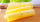A shopkeeper cuts a wheel of cheese into 10 equal wedges. A customer buys one-fifth of the wheel. How many wedges does the customer buy? Use the number line to help find the solution.
• ExpressionsLet k represent an unknown number, express the following expressions: 1. The sum of the number n and two 2. The quotient of the numbers n and nine 3. Twice the number n 4. The difference between nine and the number n 5. Nine less than the number n
• Father and daughterFather is 36 years old, daughter is 20 years less. What will be the ratio between them when they are 10 years more?
• Mineral water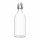The bottle contains 1.5 liters of mineral water. Pour all the water from the bottle into empty cups with a volume of 1/3 l. All but one will be filled to the brim. What part of the volume of the last cup is filled with water?
• ChestnutsThree divisions of nature protectors participated in the collection of chestnut trees.1. the division harvested 1250 kg, the 2nd division by a fifth more than the 1st division and the 3rd division by a sixth more than the second division. How many tons of
• University bubbleYou'll notice that the college up slowly every other high school. In Slovakia/Czech republic a lot of people studying political science, mass media communication, social work, many sorts of management MBA. Calculate how many times more earns clever 25-yea
• The thirdThe one-third rod is blue, one-half of the rod is red, the rest of the rod is white and measures 8 cm. How long is the whole rod?
• Pumps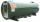6 pump fills the tank for 3 and a half days. How long will fill the tank 7 equally powerful pumps?
• Cooks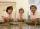Four cooks cleaned 5 kg of potatoes for 10 minutes. How many cook would have to work clean 9 kg of potatoes for 12 minutes?
• AlmondsRudi has 4 cups of almonds. His trail mix recipe calls for 2/3 cup of almonds. How many batches of trail mix can he make?
• ChocolateChildren break chocolate first to third and then every part of another half. What kind got each child? Draw a picture. What part would have received if each piece have halved?
• The balls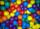You have 108 red and 180 green balls. You have to be grouped into the bags so that the ratio of red to green in each bag was the same. What smallest number of balls may be in one bag?